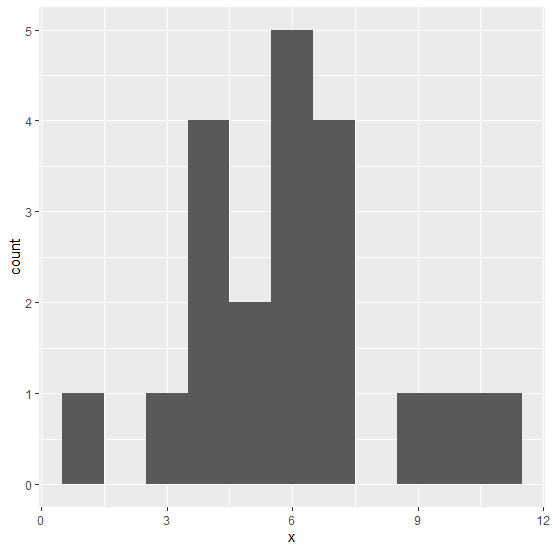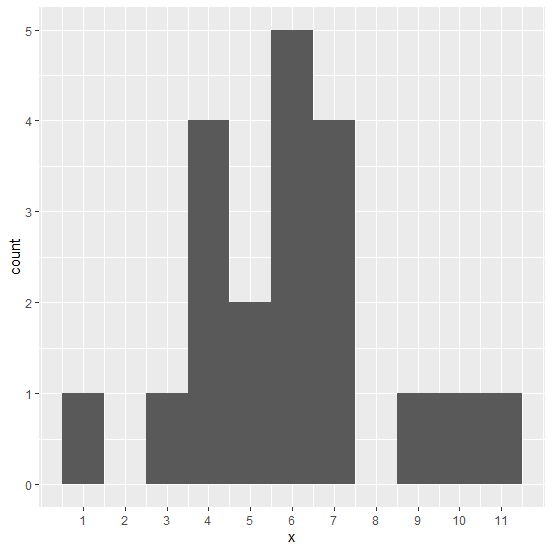# How to set the X-axis labels in histogram using ggplot2 at the center in R?

The boundary argument of geom_histogram function and breaks argument of scale_x_continuous function can help us to set the X-axis labels in histogram using ggplot2 at the center. We need to be careful about choosing the boundary and breaks depending on the scale of the X-axis values. Check out the below example to understand how it works.

## Example

Consider the below data frame −

## Example

Live Demo

> x<-rpois(20,5)
> df<-data.frame(x)
> df

## Output

x
1 5
2 7
3 6
4 4
5 7
6 7
7 10
8 3
9 6
10 6
11 5
12 4
13 4
14 6
15 7
16 4
17 1
18 11
19 6
20 9

## Example

> library(ggplot2)
> ggplot(df,aes(x))+ geom_histogram(binwidth=1)

## OutputCreating the histogram with X-axis labels at the center −

## Example

>ggplot(df,aes(x))+geom_histogram(binwidth=1,boundary=-0.5)+ scale_x_continuous(breaks=1:11)

## Output